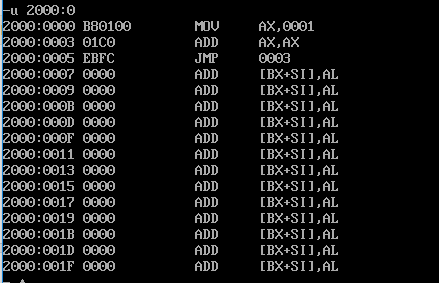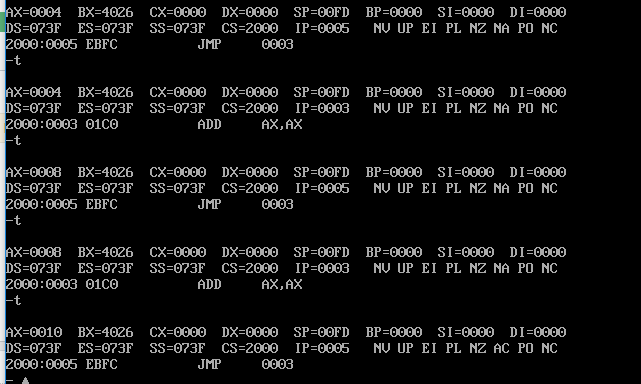3个回答

“16“用16进制表示不就是10吗

``````2^1=2DEC=0010BIN=2HEX
2^2=4DEC=0100BIN=4HEX
2^3=8DEC=1000BIN=8HEX
2^4=16DEC=10000BIN=10HEX
``````

Debug中的数值均是在十六进制形式下显示的，因此8乘以2等于十六进制的“10h”

10进制16是十六进制的10h
16进制的16h是10进制的22